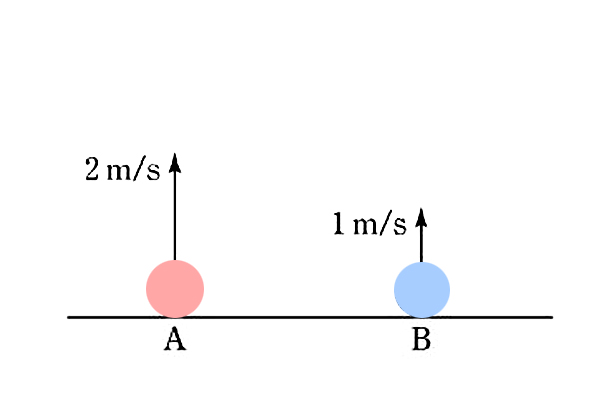# Throwing marblesTwo identical marbles $A$ and $B$ are thrown vertically from the ground with speeds of $2 \text{ m/s}$ and $1 \text{ m/s},$ respectively, as shown above. Let $a$ be the distance traveled by $A$ until it returns back to the ground. Let $b$ be the distance traveled by $B$ until it returns back to the ground. What is $\displaystyle{\frac{a}{b}?}$

×